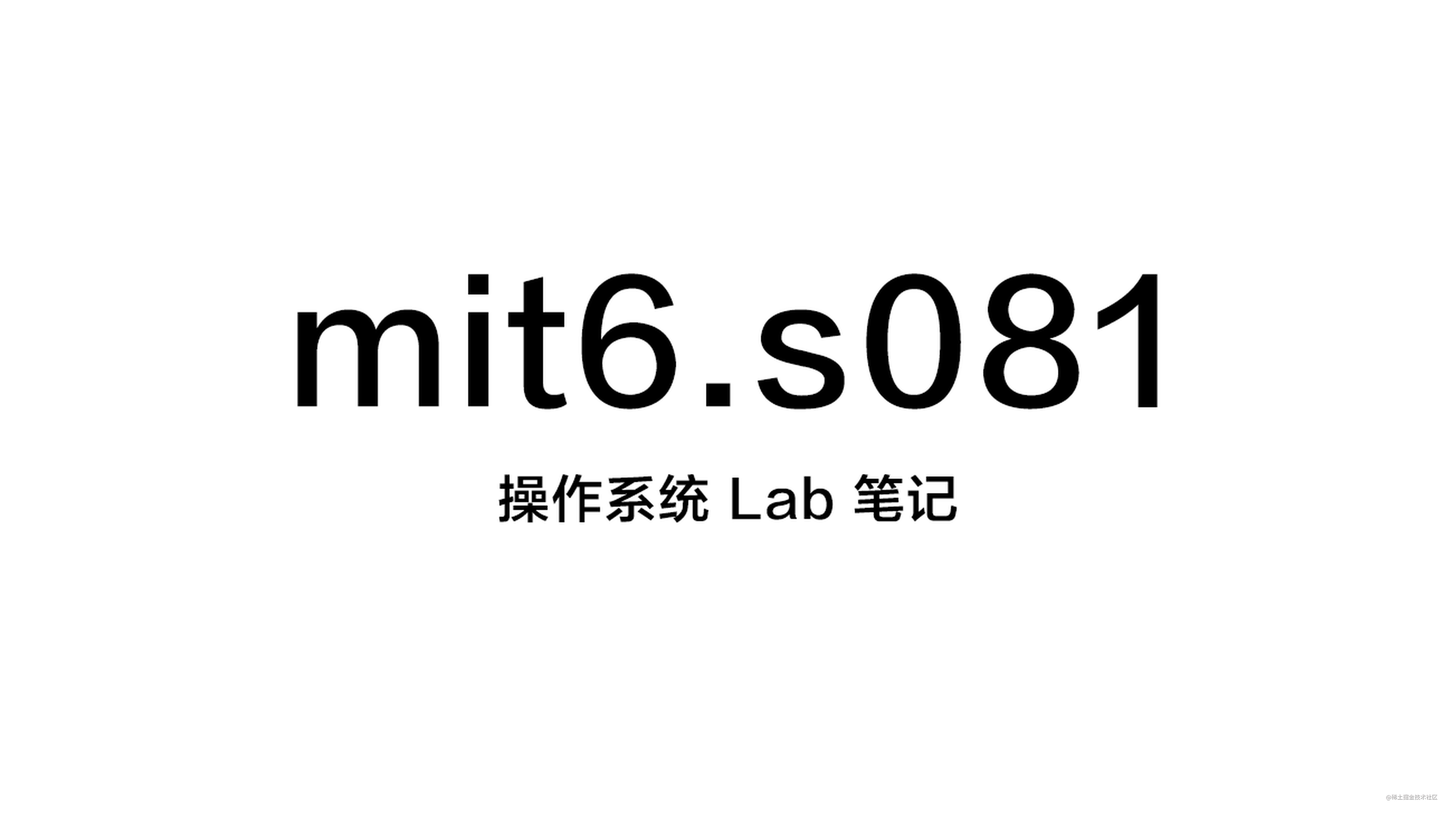# [mit6.s081] 笔记 Lab6: Copy-on-write fork | fork 懒拷贝Lab 地址：pdos.csail.mit.edu/6.S081/2020…

Commits: github.com/Miigon/my-x…

# Lab 6: Copy-on-write fork

COW fork() creates just a pagetable for the child, with PTEs for user memory pointing to the parent's physical pages. COW fork() marks all the user PTEs in both parent and child as not writable. When either process tries to write one of these COW pages, the CPU will force a page fault. The kernel page-fault handler detects this case, allocates a page of physical memory for the faulting process, copies the original page into the new page, and modifies the relevant PTE in the faulting process to refer to the new page, this time with the PTE marked writeable. When the page fault handler returns, the user process will be able to write its copy of the page.

COW fork() makes freeing of the physical pages that implement user memory a little trickier. A given physical page may be referred to by multiple processes' page tables, and should be freed only when the last reference disappears.

## Implement copy-on write (hard)

### fork 时不立刻复制内存

``````// kernel/vm.c
int
uvmcopy(pagetable_t old, pagetable_t new, uint64 sz)
{
pte_t *pte;
uint64 pa, i;
uint flags;

for(i = 0; i < sz; i += PGSIZE){
if((pte = walk(old, i, 0)) == 0)
panic("uvmcopy: pte should exist");
if((*pte & PTE_V) == 0)
panic("uvmcopy: page not present");
pa = PTE2PA(*pte);
// 清除父进程的 PTE_W 标志位，设置 PTE_COW 标志位表示是一个懒复制页（多个进程引用同个物理页）
*pte = (*pte & ~PTE_W) | PTE_COW;
flags = PTE_FLAGS(*pte);
// 将父进程的物理页直接 map 到子进程 （懒复制）
// 权限设置和父进程一致（不可写，PTE_COW）
if(mappages(new, i, PGSIZE, (uint64)pa, flags) != 0){
goto err;
}
// 将物理页的引用次数增加 1
krefpage((void*)pa);
}
return 0;

err:
uvmunmap(new, 0, i / PGSIZE, 1);
return -1;
}

``````

``````// kernel/riscv.h
#define PTE_V (1L << 0) // valid
#define PTE_R (1L << 1)
#define PTE_W (1L << 2)
#define PTE_X (1L << 3)
#define PTE_U (1L << 4) // 1 -> user can access
#define PTE_COW (1L << 8) // 是否为懒复制页，使用页表项 flags 中保留的第 8 位表示
// （页表项 flags 中，第 8、9、10 位均为保留给操作系统使用的位，可以用作任意自定义用途）
``````

### 捕获写操作并执行复制

``````// kernel/trap.c
void
usertrap(void)
{

// ......

} else if((which_dev = devintr()) != 0){
// ok
} else if((r_scause() == 13 || r_scause() == 15) && uvmcheckcowpage(r_stval())) { // copy-on-write
if(uvmcowcopy(r_stval()) == -1){ // 如果内存不足，则杀死进程
p->killed = 1;
}
} else {
printf("usertrap(): unexpected scause %p pid=%d\n", r_scause(), p->pid);
printf("            sepc=%p stval=%p\n", r_sepc(), r_stval());
p->killed = 1;
}

// ......

}
``````

``````// kernel/vm.c
int
copyout(pagetable_t pagetable, uint64 dstva, char *src, uint64 len)
{
uint64 n, va0, pa0;

if(uvmcheckcowpage(dstva))
uvmcowcopy(dstva);

// ......
}

``````

``````// kernel/vm.c
// 检查一个地址指向的页是否是懒复制页
int uvmcheckcowpage(uint64 va) {
pte_t *pte;
struct proc *p = myproc();

return va < p->sz // 在进程内存范围内
&& ((pte = walk(p->pagetable, va, 0))!=0)
&& (*pte & PTE_V) // 页表项存在
&& (*pte & PTE_COW); // 页是一个懒复制页
}

// 实复制一个懒复制页，并重新映射为可写
int uvmcowcopy(uint64 va) {
pte_t *pte;
struct proc *p = myproc();

if((pte = walk(p->pagetable, va, 0)) == 0)
panic("uvmcowcopy: walk");

// 调用 kalloc.c 中的 kcopy_n_deref 方法，复制页
// (如果懒复制页的引用已经为 1，则不需要重新分配和复制内存页，只需清除 PTE_COW 标记并标记 PTE_W 即可)
uint64 pa = PTE2PA(*pte);
uint64 new = (uint64)kcopy_n_deref((void*)pa); // 将一个懒复制的页引用变为一个实复制的页
if(new == 0)
return -1;

// 重新映射为可写，并清除 PTE_COW 标记
uint64 flags = (PTE_FLAGS(*pte) | PTE_W) & ~PTE_COW;
uvmunmap(p->pagetable, PGROUNDDOWN(va), 1, 0);
if(mappages(p->pagetable, va, 1, new, flags) == -1) {
panic("uvmcowcopy: mappages");
}
return 0;
}
``````

### 物理页生命周期以及引用计数

• kalloc(): 分配物理页
• kfree(): 释放回收物理页

• kalloc(): 分配物理页，将其引用计数置为 1
• krefpage(): 创建物理页的一个新引用，引用计数加 1
• kcopy_n_deref(): 将物理页的一个引用实复制到一个新物理页上（引用计数为 1），返回得到的副本页；并将本物理页的引用计数减 1
• kfree(): 释放物理页的一个引用，引用计数减 1；如果计数变为 0，则释放回收物理页

``````// kernel/kalloc.c

// 用于访问物理页引用计数数组
#define PA2PGREF_ID(p) (((p)-KERNBASE)/PGSIZE)
#define PGREF_MAX_ENTRIES PA2PGREF_ID(PHYSTOP)

struct spinlock pgreflock; // 用于 pageref 数组的锁，防止竞态条件引起内存泄漏
int pageref[PGREF_MAX_ENTRIES]; // 从 KERNBASE 开始到 PHYSTOP 之间的每个物理页的引用计数
// note:  reference counts are incremented on fork, not on mapping. this means that
//        multiple mappings of the same physical page within a single process are only
//        counted as one reference.
//        this shouldn't be a problem, though. as there's no way for a user program to map
//        a physical page twice within it's address space in xv6.

// 通过物理地址获得引用计数
#define PA2PGREF(p) pageref[PA2PGREF_ID((uint64)(p))]

void
kinit()
{
initlock(&kmem.lock, "kmem");
initlock(&pgreflock, "pgref"); // 初始化锁
freerange(end, (void*)PHYSTOP);
}

void
kfree(void *pa)
{
struct run *r;

if(((uint64)pa % PGSIZE) != 0 || (char*)pa < end || (uint64)pa >= PHYSTOP)
panic("kfree");

acquire(&pgreflock);
if(--PA2PGREF(pa) <= 0) {
// 当页面的引用计数小于等于 0 的时候，释放页面

// Fill with junk to catch dangling refs.
// pa will be memset multiple times if race-condition occurred.
memset(pa, 1, PGSIZE);

r = (struct run*)pa;

acquire(&kmem.lock);
r->next = kmem.freelist;
kmem.freelist = r;
release(&kmem.lock);
}
release(&pgreflock);
}

void *
kalloc(void)
{
struct run *r;

acquire(&kmem.lock);
r = kmem.freelist;
if(r)
kmem.freelist = r->next;
release(&kmem.lock);

if(r){
memset((char*)r, 5, PGSIZE); // fill with junk
// 新分配的物理页的引用计数为 1
// (这里无需加锁)
PA2PGREF(r) = 1;
}

return (void*)r;
}

// Decrease reference to the page by one if it's more than one, then
// allocate a new physical page and copy the page into it.
// (Effectively turing one reference into one copy.)
//
// Do nothing and simply return pa when reference count is already
// less than or equal to 1.
//
// 当引用已经小于等于 1 时，不创建和复制到新的物理页，而是直接返回该页本身
void *kcopy_n_deref(void *pa) {
acquire(&pgreflock);

if(PA2PGREF(pa) <= 1) { // 只有 1 个引用，无需复制
release(&pgreflock);
return pa;
}

// 分配新的内存页，并复制旧页中的数据到新页
uint64 newpa = (uint64)kalloc();
if(newpa == 0) {
release(&pgreflock);
return 0; // out of memory
}
memmove((void*)newpa, (void*)pa, PGSIZE);

// 旧页的引用减 1
PA2PGREF(pa)--;

release(&pgreflock);
return (void*)newpa;
}

// 为 pa 的引用计数增加 1
void krefpage(void *pa) {
acquire(&pgreflock);
PA2PGREF(pa)++;
release(&pgreflock);
}
``````

``````父进程: 分配物理页 p（p 引用计数 = 1）

--- 调度器切换到子进程

--- 调度器切换到父进程

``````

### 执行测试

``````\$ make grade
......
== Test running cowtest ==
\$ make qemu-gdb
(10.4s)
== Test   simple ==
simple: OK
== Test   three ==
three: OK
== Test   file ==
file: OK
== Test usertests ==
\$ make qemu-gdb
(99.8s)
== Test   usertests: copyin ==
usertests: copyin: OK
== Test   usertests: copyout ==
usertests: copyout: OK
== Test   usertests: all tests ==
usertests: all tests: OK
== Test time ==
time: OK
Score: 110/110
``````

``````FAILED -- lost some free pages 32442 (out of 32448)
``````